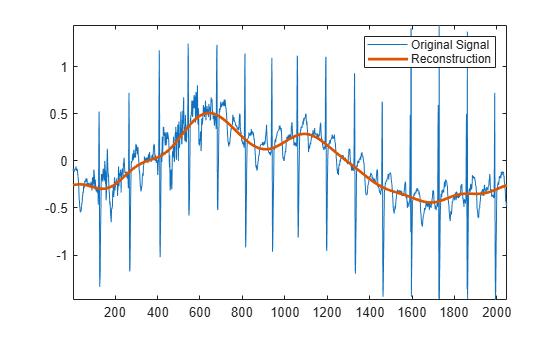# itqwt

Inverse tunable Q-factor wavelet transform

## Syntax

``xrec = itqwt(wt,n)``
``xrec = itqwt(wt,n,Name=Value)``

## Description

````xrec = itqwt(wt,n)` returns the inverse tunable Q-factor transform (TQWT) of the analysis coefficients in `wt`. `wt` is a cell array containing the wavelet subband and lowpass coefficients obtained from `tqwt` using the default quality factor of 1. `n` is the original signal length specified as a positive scalar.```

example

````xrec = itqwt(wt,n,Name=Value)` specifies one or more additional name-value arguments. For example, ```xrec = itqwt(x,1024,QualityFactor=2)``` specifies a quality factor of 2.```

## Examples

collapse all

Load an ECG signal. Obtain the tunable Q-factor wavelet transform to the default maximum decomposition level using a quality factor of 3.

```load wecg qf = 3; wt = tqwt(wecg,QualityFactor=qf);```

Reconstruct the data from the subband coefficients and confirm perfect reconstruction.

```xrec = itqwt(wt,length(wecg),QualityFactor=qf); max(abs(xrec-wecg))```
```ans = 5.5511e-16 ```

Return the inverse TQWT at the coarsest scale.

```xrec = itqwt(wt,length(wecg),QualityFactor=qf,Level=length(wt)-2); plot(wecg) hold on plot(xrec,LineWidth=2) hold off axis tight legend("Original Signal","Reconstruction")```## Input Arguments

collapse all

Tunable Q-factor wavelet transform, specified as a cell array. The elements of `wt` contain the wavelet subband and lowpass coefficients. `wt` is expected to be the output of `tqwt`.

Data Types: `single` | `double`
Complex Number Support: Yes

Original signal length in samples, specified as a positive integer. If `n` is odd, `n+1` is used internally to invert the TQWT and the first `n` samples are returned.

Data Types: `single` | `double`

### Name-Value Arguments

Specify optional pairs of arguments as `Name1=Value1,...,NameN=ValueN`, where `Name` is the argument name and `Value` is the corresponding value. Name-value arguments must appear after other arguments, but the order of the pairs does not matter.

Example: `xrec = itqwt(wt,2048,Level=3,QualityFactor=2)`

Reconstruction level of analysis coefficients, specified as a nonnegative integer less than or equal to `length(wt)-2`. If unspecified, `itqwt` reconstructs the TQWT up to the resolution level of the original signal.

Example: `xrec = itqwt(x,Level=1)` returns the inverse TQWT at level 1.

Data Types: `single` | `double`

Quality factor, specified as a positive scalar greater than or equal to 1. The quality factor must match the value used in the TQWT.

Example: `xrec = itqwt(x,QualityFactor=2)` specifies a quality factor of 2.

Data Types: `single` | `double`

## Output Arguments

collapse all

Inverse tunable-Q wavelet transform, returned as a vector, matrix, or 3-D array.

Data Types: `single` | `double`

## Tips

• To reconstruct only the scaling coefficients, specify a reconstruction level of `length(wt)-1`.

 Selesnick, Ivan W. “Wavelet Transform With Tunable Q-Factor.” IEEE Transactions on Signal Processing 59, no. 8 (August 2011): 3560–75. https://doi.org/10.1109/TSP.2011.2143711.